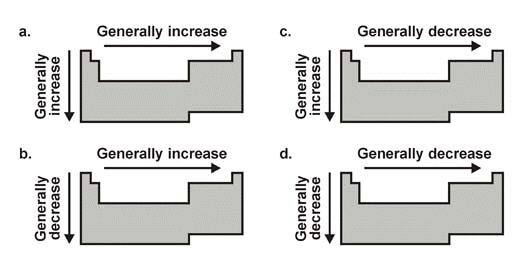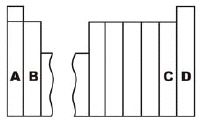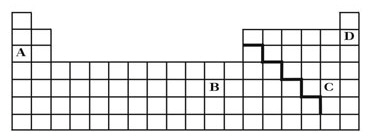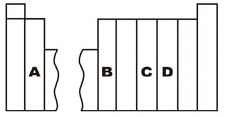﻿ Unit 2 Praactice Test - Periodic Table
 Name:    Chapter 5 Practice Test - Periodic Table

Multiple Choice
Identify the choice that best completes the statement or answers the question.

1.

A negative ion is known as a(n)
 a. cation. b. anion c. subion d. cassion

2.

Hydrogen is placed separately from other elements in the periodic table because it
 a. has many unique properties and is unlike any other element. b. is radioactive. c. does not exist in nature. d. it is not really an element.

3.

The energy required to remove an electron from an atom while it is bonded?

4.

Which is the correct number of the valence shell for the element Gallium (Ga)?
 a. 4 b. 13 c. 3 d. 25.

Which diagram correctly depicts the general trend in atomic radius?
 a. a b. b c. c d. d

6.

Which diagram correctly depicts the general trend in ionization energy?
 a. a b. b c. c d. d

7.

Which diagram correctly depicts the trend in electronegativity?
 a. a b. b c. c d. d

8.

Which is the correct number of valence electrons in the element Gallium (Ga)?
 a. 13 b. 4 c. 3 d. 29.

Which region is referred to as the f-block on the diagram?
 a. A b. B c. C d. D

10.

Which region is referred to as the p-block on the diagram?
 a. A b. B c. C d. D11.

Which region contains the halogen family of elements?
 a. A b. B c. C d. D

12.

Which region contains the alkali metal family of elements?
 a. A b. B c. C d. D

13.

Which region contains the noble gas family of elements?
 a. A b. B c. C d. D

14.

Which region contains the alkaline earth metal family of elements?
 a. A b. B c. C d. D

15.

The measure of an atoms attraction for electrons is known as:
 a. electronegativity. c. electron energy. b. electron affinity. d. ionization energy.16.

Which element has an electron configuration that ends with s1?
 a. A b. B c. C d. D

17.

Which element loses ONE electron to be like a noble gas?
 a. A b. B c. C d. D

18.

Which element has an electron configuration that ends with its valence shell completely filled?
 a. A b. B c. C d. D

19.

Mendeleev created a periodic table of the elements that repeated at regular intervals when the elements were arranged in order of increasing
 a. reactivity. c. atomic number. b. atomic mass. d. density.20.

Which region contains elements that gain two electrons to become 2- ions??
 a. A b. B c. C d. D

21.

Which region contains elements with two valence electrons?
 a. A b. B c. C d. D

22.

Mendeleev predicted that the spaces in his periodic table represented
 a. radioactive elements. c. unstable elements. b. isotopes. d. undiscovered elements.

23.

Which group will have an electron configuration that ends in s2?
 a. Alkaline Earth metals c. Alkali metals b. Actinides d. Halogens

24.

Which scientist arranged elements into “octaves”?
 a. Dobereiner b. Mendeleev c. Mosely d. Newlands

25.

The most characteristic property of the noble gases is that they
 a. have low boiling points. b. are largely unreactive. c. are radioactive. d. are gases at ordinary temperatures.

26.

The quanta of light that is given off by electrons changing energy levels is knows a
 a. neon b. boson c. photon d. quark

27.

Which of the following elements is a metal?
 a. C b. N c. B d. Mg

28.

A horizontal row of blocks in the periodic table is called a(n)
 a. family. b. period. c. octet. d. group.

29.

The element that has the greatest electronegativity is
 a. sodium. Na b. fluorine. F c. oxygen. O d. chlorine. Cl

30.

In which pair is the one on the left larger than the one on the right? ( L > R )
 a. K, K+ c. neither of these b. Br, Br- d. both of these

31.

Emission of light from an atom occurs when an electron ____.
 a. moves within its atomic orbital b. jumps from a lower to a higher energy level c. drops from a higher to a lower energy level d. falls into the nucleus

32.

The most reactive element of the nonmetals (excluding hydrogen and the noble gases)
 a. Na b. H c. F d. Fr

33.

Which scientist first arranged elements by atomic number?
 a. Mendeleev b. Dobereiner c. Newlands d. Mosely

34.

Which is the halogen that is in Period 5?
 a. Iodine (I) b. Bromine (Br) c. Xenon (Xe) d. Strontium (Sr)

35.

Which correctly describes elements that a located in the same group?
 a. They must be in the same state of matter. b. They have the same number of valence electrons. c. They have the same atomic radius. d. They have electrons in the same valence shell.